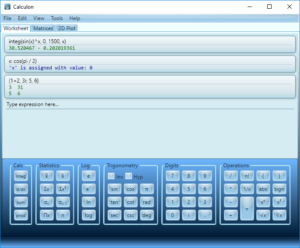# Calculon Overview

Powerful, lightweight complex system for mathematical calculations, which brings to you the opportunity to solve your problems, as fast as possible. More than 160 built-in functions from different areas of math, full support of complex numbers and matrix calculations (even complex matrix calculations). The system itself contains a wide range of numerical methods, such as numeric integration, finding roots, solving linear systems and much more.The application supports numbers in different formats: decimal, binary, octal, hexadecimal (10, 1010b, 12o, 0Ah).

Embedded help system which contains samples of application usage, full list of functions with descriptions and examples.

Existing three working modes:

• Worksheet view – an interactive mathematical console with a history of calculations, ability to define variables, etc.
• Matrices view – table worksheet which was designed primarily for matrix calculations.
• Plot 2D view – plot for drawing functions in Cartesian coordinates.

Beautiful, user-friendly interface with the ability to change the color scheme and fully support the High DPI displays.

## Key Features

160+ built-in math functions

• High-quality numeric methods
• Defining variables
• Сalculations history
• Stylized formatting of results
• Excel-like matrix worksheet
• Drawing graphs of functions
• Support different types of numbers: decimal, binary, octal and hexadecimal
• Different UI color schemes
• Full support of High DPI displays
• References help